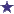# IV Question on Nitroprusside 50mg in 250ml of D5WMedication:

Nitroprusside 50mg in 250mL D5W

Dose: 5mcg/kg/min

Patient weight: 143lb

A: How many milliliters per hour?

B: How many milligrams per hour?

### Comments for IV Question on Nitroprusside 50mg in 250ml of D5W

Average RatingRatingCorrected Answer **Please Double check me** by: Barry 143lbs/2.2kg/lb=65kg 5mcg/kg/min 5mcg*65kg=325mcg/min Now convert micrograms to milligrams. 325mcg/min divided by 1000=0.325mg/min Now we can figure how many milligrams per hour: 0.325mg/min*60min/1hr=19.5mg/hr (see question B, below) A: How many milliliters per hour? 200mcg/ml 19.5mg/hr Convert 19.5mg/hr to micrograms to have the same units: 0.0195mcg/hr divided by 200mcg/ml 97.5ml/hr B: How many milligrams per hour? 19.5mg/hr

 RatingWrong by: Anonymous People the calculation for kilograms is not right. Running that much of nitroprusside in the an hour would kill the patient in a matter of minutes. Plus look at the amount of fluid going in over an hour. Cardiac patient and fluid overload equals bad patient outcome. To find kilograms it's lbs ÷2.2=kilograms. Look at your answers and think about the patient outcome.... a drip is a small amount over a period of time.

 RatingAnswer: Millileters Per Hour and Milligrams Per Hour by: Brandon First, look at the information we have.Dose: 5mcg/kg/minandPatient weight: 143 lbWe have to convert pounds to kilograms. We do this by multiplying 2.2 (there are 2.2 kilograms in each pound) by 143 lb, which equals 314.6 kilograms.So now we figure the dose. We multiply 314.6 by 5mcg and we get 1,573 mcg/min.Now we need to convert micrograms to milligrams. There are 1000 micrograms in 1 milligram. So 1,573 mcg/min = 1.573 mg/minSo, going back to our questions: (We will answer the second question first.) How many milligrams per hour?To get milligrams per hour, we take our last answer, 1.573 mg/min and multiply it by 60 (60 min / 1 hour), which equals 94.38 mg/hr.So back to the first question: How many milliliters per hour?Using assumptions from the documentation/package insert on Nipride, there are 200 micrograms/mlWe have mg/hr (94.38 mg/hr from last question.) Also, we have 1573 mcg/min from the last question. Now we find milliliters per minute:Divide 1573 mcg/min by 200 mcg/ml = 7.865 milliliters per minuteThen, to get the answer, we multiply 7.865 milliliters/minute by 60 (sixty minutes in 1 hour) and we get 471.9 milliliters per hour